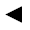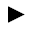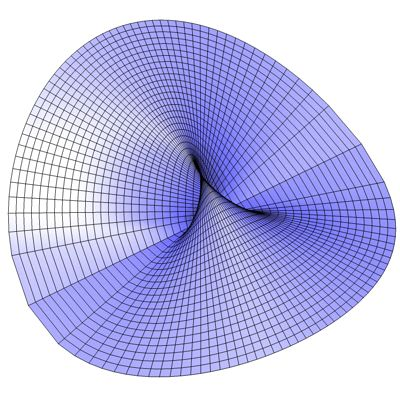[back]

## 3.11. Plücker's Conoid

[next]The Plücker Conoid [ 6,11,18 ] is represented by the following equations.

 x = u sqrt(1 - v 2 ) 3-45 y = u v 3-46 z = 1 - v 2 3-47

To represent the area, the two parameters u and v can assume the following values ​​(domain of definition), for example.

 u is an element from the set of numbers [-2, 2] v is an element from the number set [-1, 1]

The Plücker's Conoid is an open figure, so the definition range can be changed with the plugin.Fig. 15